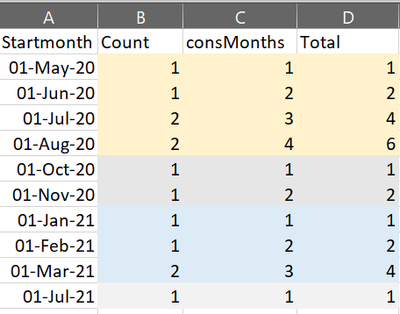cancel
Showing results for
Did you mean:Regular Visitor

## Total sum for consecutive period

Hello Dax gurus!,

I have the count for each consecutive month  in the below screen shot. What i want to get is the values in the Total column. For each consMonth 1,2,3,4....., i want to add up the values in the count column.
May 20 = 1

Jun 20 = 1+1

July 20 = 1 + 1 + 2

Aug and oct or not consecutive months so the total start again in Oct and so on. Here is the output i am expecting:thanks

3 REPLIES 3Solution Sage

Hi,

``````Total =
VAR ThisStartMonth = 'Table'[Startmonth]
VAR FirstMonth =
CALCULATE (
MAX ( 'Table'[Startmonth] ),
FILTER (
'Table',
'Table'[Startmonth] <= ThisStartMonth
&& 'Table'[consMonths] = 1
)
)
RETURN
CALCULATE (
SUM ( 'Table'[Count] ),
FILTER (
'Table',
'Table'[Startmonth] >= FirstMonth
&& 'Table'[Startmonth] <= ThisStartMonth
)
)``````

RegardsSuper User

@aristotle I think you are going to have to start with a resetting counter and that is Cthulhu:

Cthulhu - Microsoft Power BI Community

Become an expert!: Enterprise DNA
External Tools: MSHGQM
Latest book!:
Mastering Power BI 2nd EditionDAX is easy, CALCULATE makes DAX hard...Solution Sage

@Greg_Deckler Love the choice of name! 😀